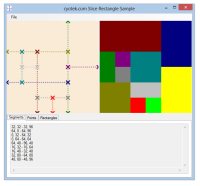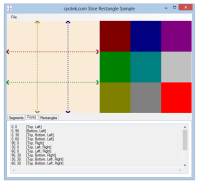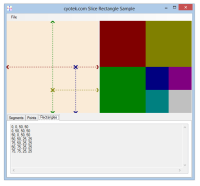## Dividing up a rectangle based on pairs of points using C#

Recently we released the first alpha of our latest product, Cyotek Slicr, a tool for slicing up an image. At the heart of this tool is a series of routines that take a given image and pairs of input points, from which the image is chopped up accordingly. This article describes how to break up a rectangle into smaller parts based on user defined co-ordinates.### Caveat Emptor

Before I get started, I just want to point out that the code I'm about to show you is proof of concept code. It doesn't use algorithms such as Bentley–Ottmann, it's not very efficient, and there's probably a hundred ways of doing it better. However, it works, which is pretty much all I care about at this moment!

### Getting Started

These are the rules for splitting up a rectangle into component parts:

1. Lines must be straight, so each pair of co-ordinates will align on one axis
2. Co-ordinates must start from either an edge, or the intersection of another pair. The second co-ordinate must end in a similar fashion. Any "orphan" co-ordinates which don't start/end on another edge/join are illegal and should be ignored
3. Co-ordinates can start and end at any point in the bounds of the canvas, as long as they follow the previous rules.

In order to achieve this, I ended up with creating a number of support classes

• `Segment` - this class starts the starting point of a line, it's length, and it's orientation. Originally I just started by storing the two pairs of co-ordinates, but in the end it was easier with the length and orientation.
• `SegmentPoint` - this class stores the details of a single point. An instance of this class is created for each unique point created by the start and end of a segment, and any intersections. It also stores the directions of neighbouring points.
``````internal class Segment
{
public Point EndLocation
{
get
{
int x;
int y;

switch (this.Orientation)
{
case SegmentOrientation.Vertical:
x = this.Location.X;
y = this.Location.Y + this.Size;
break;
default:
x = this.Location.X + this.Size;
y = this.Location.Y;
break;
}

return new Point(x, y);
}
}

public Point Location { get; set; }

public SegmentOrientation Orientation { get; set; }

public int Size { get; set; }
}

internal class SegmentPoint
{
public SegmentPointConnections Connections { get; set; }

public Point Location { get; set; }

public int X { get { return this.Location.X; } }

public int Y { get { return this.Location.Y; } }
}``````

With these helper classes, I can now construct the code to process them and extract the rectangles.

### Calculating PointsThe image above demonstrates the first problem. The four segments intersect with each other, causing a rectangle to be generated untouched by any user defined point. However, if we are going to find that rectangle, we need to find any new point generated by multiple segments intersecting.

``````private void CalculatePoints()
{
List<Segment> segments;

segments = new List<Segment>();
this.Points = new Dictionary<Point, SegmentPoint>();

// add segments representing the edges
segments.Add(new Segment { Location = Point.Empty, Size = this.Size.Width, Orientation = SegmentOrientation.Horizontal });
segments.Add(new Segment { Location = new Point(0, this.Size.Height), Size = this.Size.Width, Orientation = SegmentOrientation.Horizontal });
segments.Add(new Segment { Location = Point.Empty, Size = this.Size.Height, Orientation = SegmentOrientation.Vertical });
segments.Add(new Segment { Location = new Point(this.Size.Width, 0), Size = this.Size.Height, Orientation = SegmentOrientation.Vertical });

// add the rest of the segments

segments.Sort((a, b) =>
{
int result = a.Location.X.CompareTo(b.Location.X);
if (result == 0)
result = a.Location.Y.CompareTo(b.Location.Y);
return result;
});

foreach (Segment segment in segments)
{
Segment currentSegment;

this.UpdatePoint(segment.Location, segment.Orientation == SegmentOrientation.Horizontal ? SegmentPointConnections.Left : SegmentPointConnections.Top);
this.UpdatePoint(segment.EndLocation, segment.Orientation == SegmentOrientation.Horizontal ? SegmentPointConnections.Right : SegmentPointConnections.Bottom);

// calculate any intersecting points
currentSegment = segment;
foreach (Segment otherSegment in segments.Where(s => s != currentSegment))
{
Point intersection;

intersection = Intersection.FindLineIntersection(segment.Location, segment.EndLocation, otherSegment.Location, otherSegment.EndLocation);
if (!intersection.IsEmpty)
{
SegmentPointConnections flags;

flags =  SegmentPointConnections.None;
if (intersection != segment.Location && intersection != segment.EndLocation)
{
if (segment.Orientation == SegmentOrientation.Horizontal)
flags |= ( SegmentPointConnections.Left | SegmentPointConnections.Right);
else
flags |= (SegmentPointConnections.Top | SegmentPointConnections.Bottom);
}
else if (intersection != otherSegment.Location && intersection != otherSegment.EndLocation)
{
if (otherSegment.Orientation == SegmentOrientation.Horizontal)
flags |= (SegmentPointConnections.Left | SegmentPointConnections.Right);
else
flags |= (SegmentPointConnections.Top | SegmentPointConnections.Bottom);
}

if (flags != SegmentPointConnections.None)
this.UpdatePoint(intersection, flags);
}
}
}
}``````

Breaking the code down, we do the following:

• Create an additional four segments representing the boundaries of the canvas
• Sort the segments by their starting locations
• Cycle each segment and
• Create a point for the starting co-ordinate
• Create a point for the ending co-ordinate
• Cycle each other segment and see if it intersects with the current segment. If it does, create a new point at the intersection

In any case above where I state to create a point, the code will either create a point if one doesn't already exist, otherwise it will update the connections of the existing point.

``````private void UpdatePoint(Point location, SegmentPointConnections connections)
{
SegmentPoint point;

if (!this.Points.TryGetValue(location, out point))
{
point = new SegmentPoint { Location = location, Connections = connections };
}
else if (!point.Connections.HasFlag(connections))
point.Connections |= connections;
}``````

The `CalculatePoints` method above is very inefficient, but it does the job. Once this routine has run, we'll have an array of co-ordinates and the directions of linked points.

### Calculating Rectangles

Now that we have all points, both user defined, and intersections, we can use this to generate the actual rectangles.

``````private void CalculateRectangles()
{
SegmentPoint[] horizontalPoints;
SegmentPoint[] verticalPoints;

this.Rectangles = new HashSet<Rectangle>();
horizontalPoints = this.Points.Values.OrderBy(p => p.X).ToArray();
verticalPoints = this.Points.Values.OrderBy(p => p.Y).ToArray();

foreach (SegmentPoint topLeft in this.Points.Values.Where(p => p.Connections.HasFlag(SegmentPointConnections.Left | SegmentPointConnections.Top)))
{
SegmentPoint topRight;
SegmentPoint bottomLeft;

topRight = horizontalPoints.FirstOrDefault(p => p.X > topLeft.X && p.Y == topLeft.Y && p.Connections.HasFlag(SegmentPointConnections.Right | SegmentPointConnections.Top));
bottomLeft = verticalPoints.FirstOrDefault(p => p.X == topLeft.X && p.Y > topLeft.Y && p.Connections.HasFlag(SegmentPointConnections.Left | SegmentPointConnections.Bottom));

if (topRight != null && bottomLeft != null)
{
SegmentPoint bottomRight;

bottomRight = horizontalPoints.FirstOrDefault(p => p.X == topRight.X && p.Y == bottomLeft.Y && p.Connections.HasFlag(SegmentPointConnections.Right | SegmentPointConnections.Bottom));

if (bottomRight != null)
{
Rectangle rectangle;

rectangle = new Rectangle(topLeft.X, topLeft.Y, bottomRight.X - topLeft.X, bottomRight.Y - topLeft.Y);

}
}
}
}``````

In this method, we loop through all our defined points that have connections in the upper left corner.

For each matching point, we then try and find points with the following criteria

• has the same Y co-ordinate and a right or top connection. This gives us the upper right corner.
• has the same X co-ordinate and a left or bottom connection. This gives us the lower left corner.
• if we have both the above corners, we then try and find a point that has the same X co-ordinate as the upper right corner, the same Y co-ordinate as the lower left corner, and right or bottom connections. This gives us the last corner, lower right
• if we have all four corners, and the rectangle. The use of a `HashSet` ensures the same rectangle isn't added twice.

And that's all there is to it. With these two routines, I can now break up a rectangle into many smaller pieces just by defining pairs of points.### Closing Remarks

There are a few things that I'm aware of that the code doesn't do

• As mentioned (several times!) none of this code is particularly efficient. The more segments you add, the slower it will get. Gareth Rees posted a nice diagram of what I should be doing, and indeed his pointers help me get this code working originally.
• It doesn't handle overlapping segments very well. It will rerun the point generation for these, adding to the overall time.
• Ordering of the output rectangles isn't always what you expect - it jumps around a bit
• The end coordinate must be equal to or greater than the start (using the sample, providing a negative segment size would trigger this bug).

Filename Description Version Release Date
SliceRectangleSample.zip
• md5: 1e8ccdf10305cc43789e4fa28c96006b

An sample project which demonstrates how to use C# to split a rectangle into multiple smaller parts based on pairs of co-ordinates.

10/02/2013

While we appreciate comments from our users, please follow our posting guidelines. Have you tried the Cyotek Forums for support from Cyotek and the community?

Styling with Markdown is supported